# Spatiotemporal ResNet-18 for Action Recognition Trained onKinetics-400 Data

Identify the main action in a video

Released in 2018, this family of models is obtained by splitting the 3D convolutional filters into distinct spatial and temporal components yielding a significant increase in accuracy.

Number of models: 3

## Training Set Information

• Kinetics-400 Human Action Video Data, consisting of four hundred human action classes, with at least four hundred video clips for each action. Each clip lasts around 10 seconds and is taken from a different YouTube video.

## Performance

• The models achieve the following 5-crop accuracies for clip length 16 (16×112×112) on the Kinetics-400 dataset.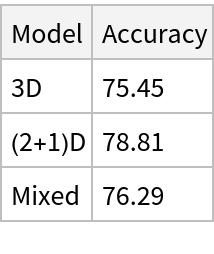## Examples

### Resource retrieval

Get the pre-trained net:

 In:=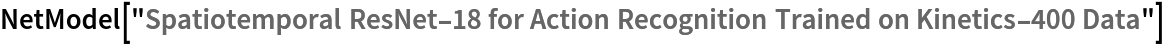Out=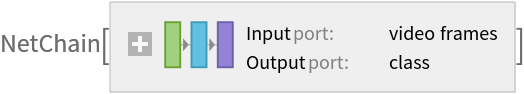### NetModel parameters

This model consists of a family of individual nets, each identified by a specific parameter combination. Inspect the available parameters:

 In:=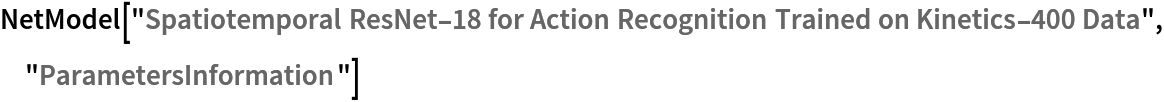Out=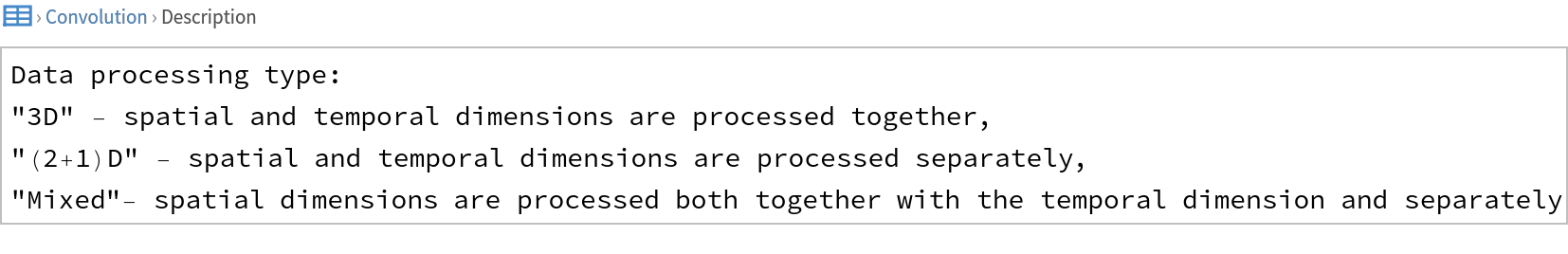Pick a non-default net by specifying the parameters:

 In:=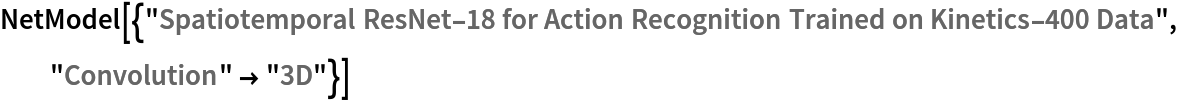Out=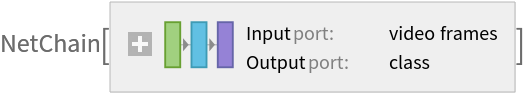Pick a non-default uninitialized net:

 In:=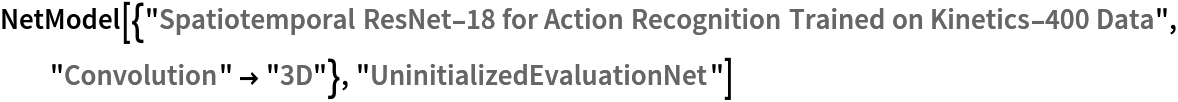Out=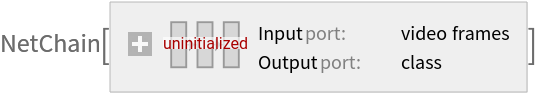### Basic usage

Get a video:

 In:=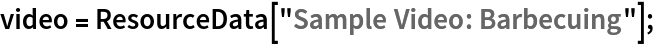Show some of the video frames:

 In:=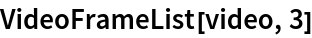Out=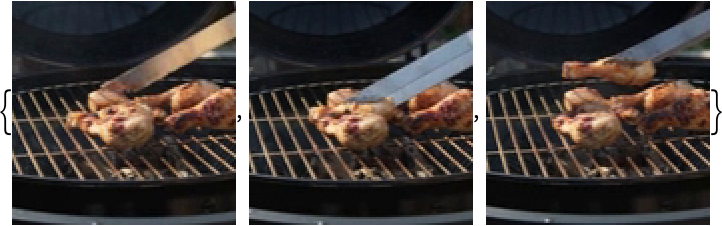Identify the main action in a video:

 In:=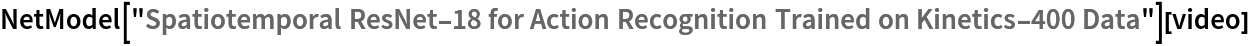Out=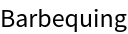Obtain the probabilities of the 10 most likely entities predicted by the net:

 In:=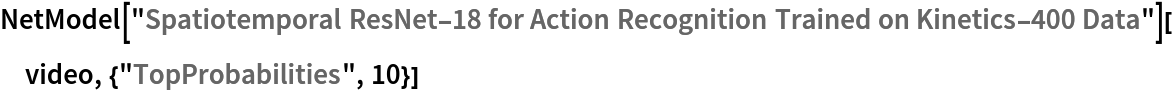Out=An activity outside the list of the Kinetics-400 classes will be misidentified:

 In:=Out=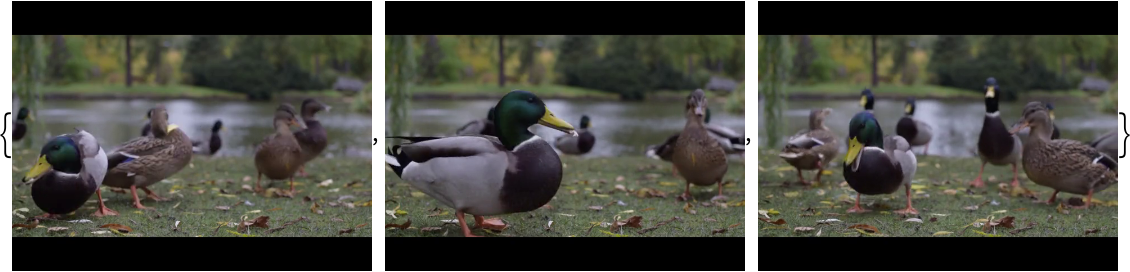In:=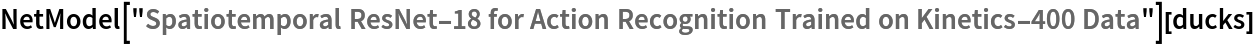Out=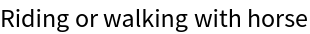Obtain the list of names of all available classes:

 In:=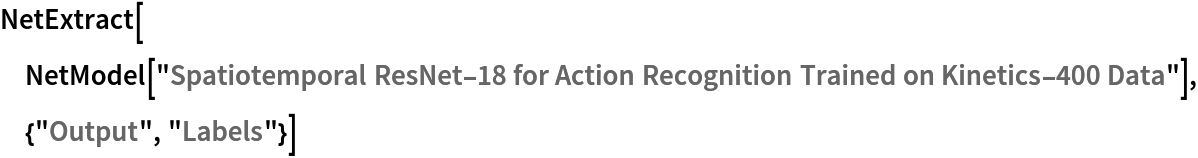Out=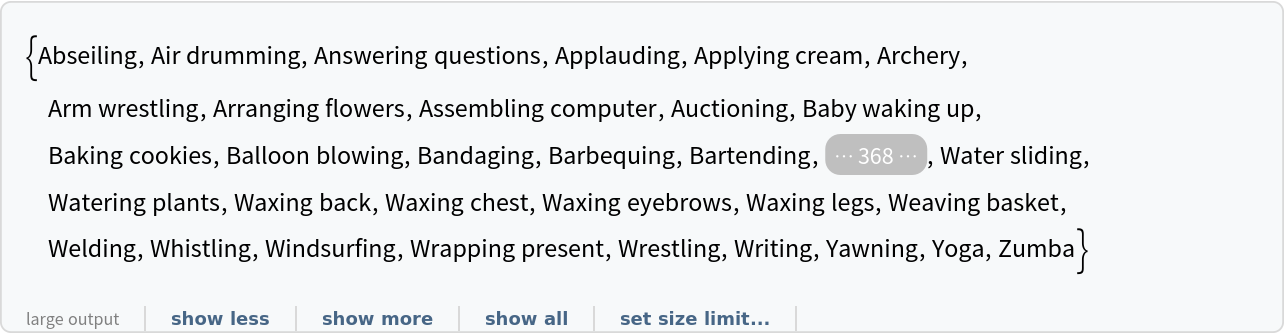Identify the main action of the video over the moving frames:

 In:=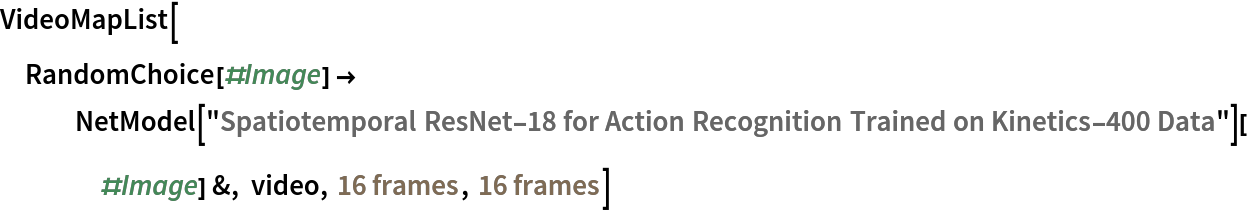Out=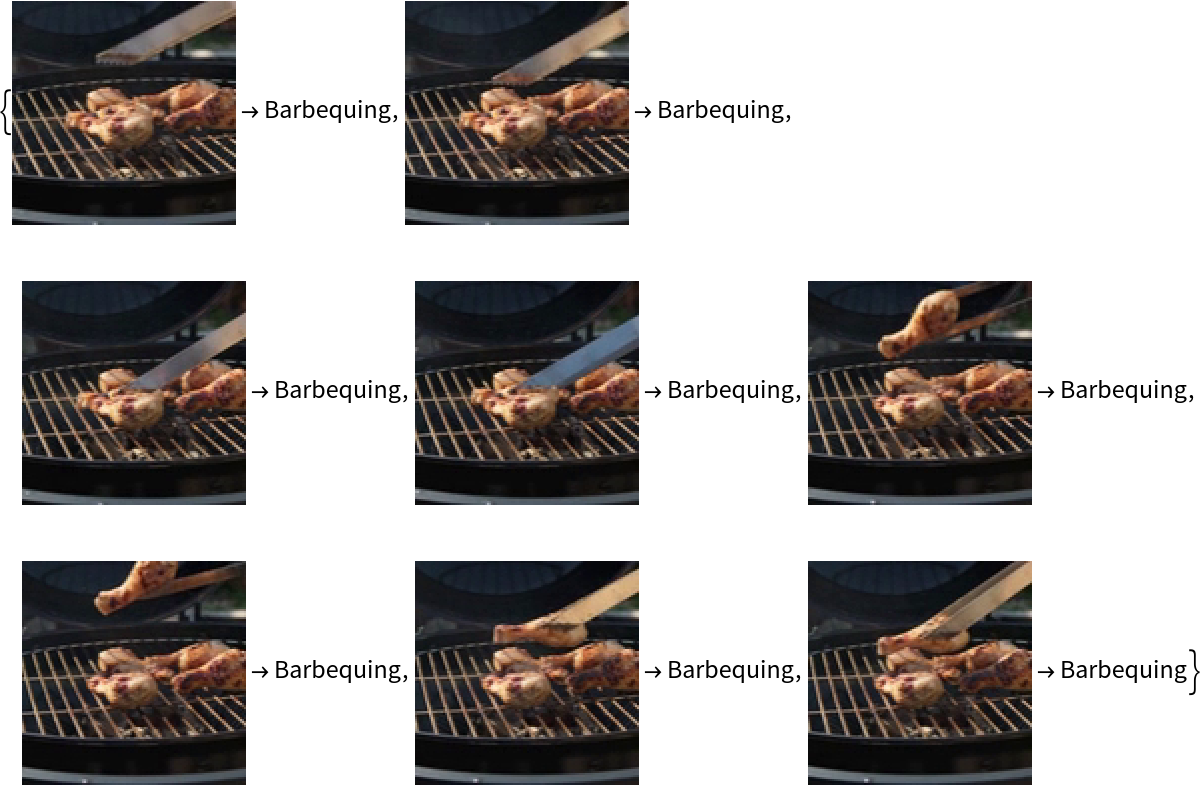### Visualize convolutional weights

Extract the weights of the first convolutional layer in the trained net:

 In:=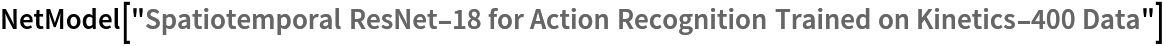Out=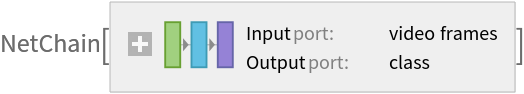In:=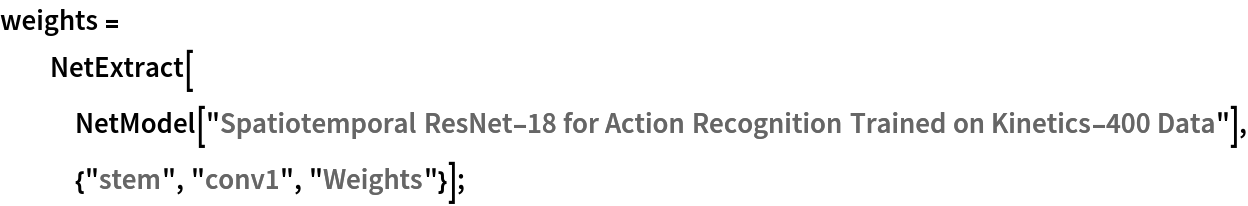Show the dimensions of the weights:

 In:=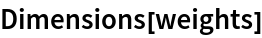Out=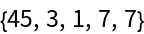Extract the kernels corresponding to the receptive fields:

 In:=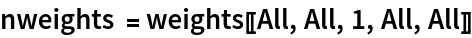Out=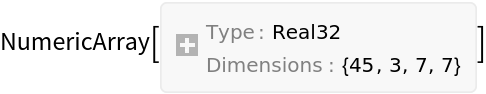Visualize the weights as a list of 45 images of size 7⨯7:

 In:=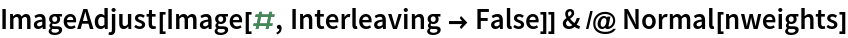Out=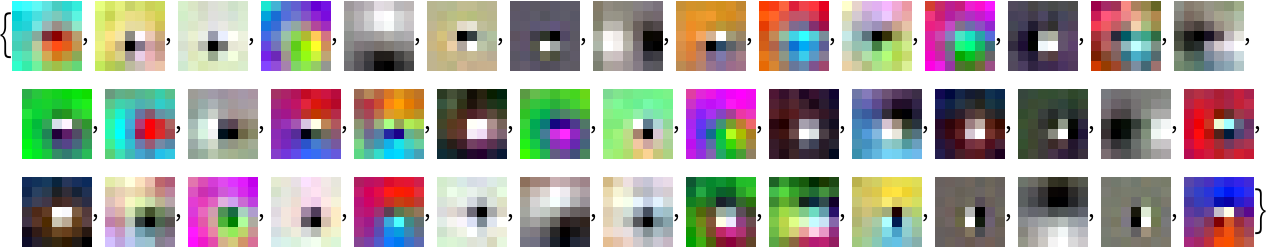### Network architecture

3D convolutional neural networks (3DCNNs) preserve temporal information, with filters over both time and space. "3D" architecture has all the convolutional blocks as 3DCNN. 3D kernels have a kernel size of L×H×W, where L denotes the temporal extent of the filter and H×W are the height and width of the filter. Extract the kernel size of the first convolutional layer from each of the convolutional blocks:

 In:=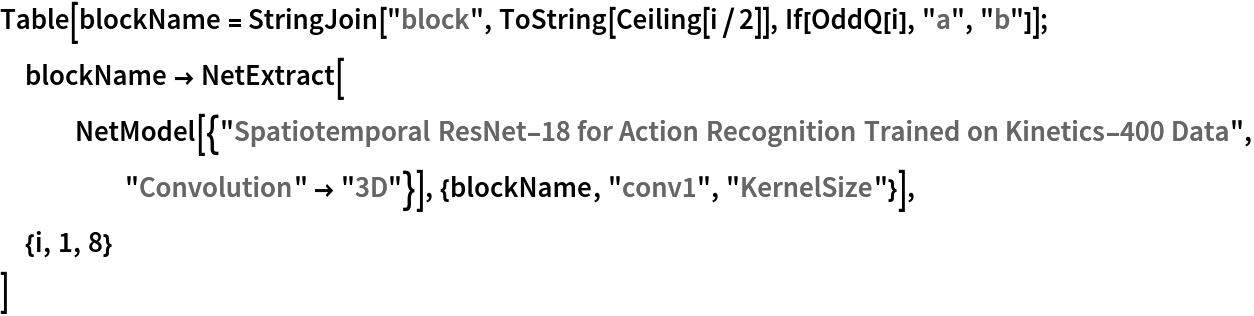Out="Mixed" 3D convolutional neural networks (3DCNNs) use 3D convolutions for early layers and 2D convolutions for later layers. In the later blocks, L=1, which implies that different frames are processed independently:

 In:=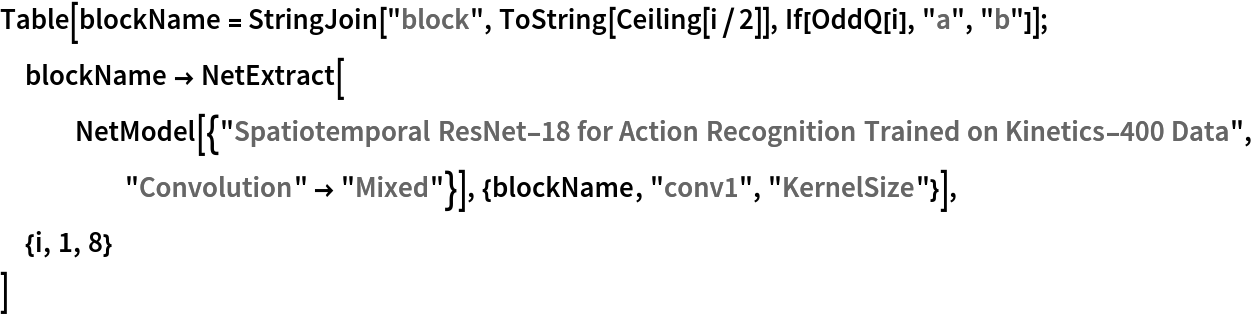Out=Another way to approach this problem would be to replace 3D kernels of size L×H×W with a "(2+1)D" block consisting of spatial 2D convolutional filters of size 1×H×W and temporal convolutional filters of size L×1×1. Extract the first two convolution kernels from each block to explore the alternate spatial and temporal convolutions:

 In:=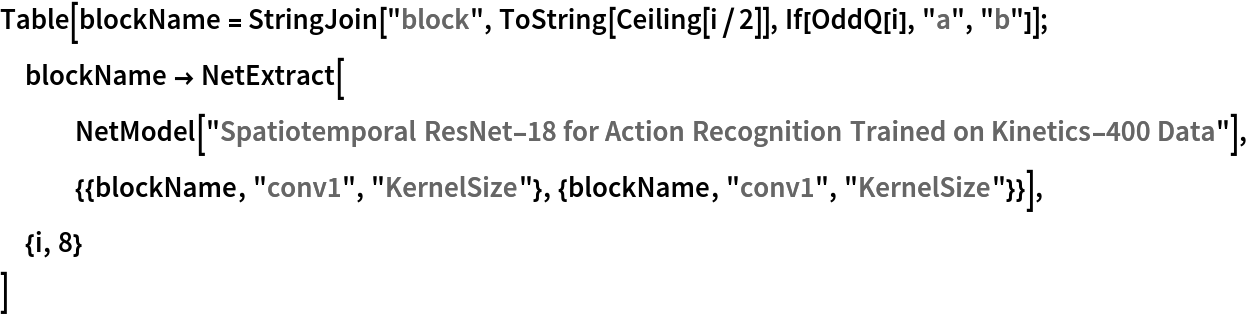Out=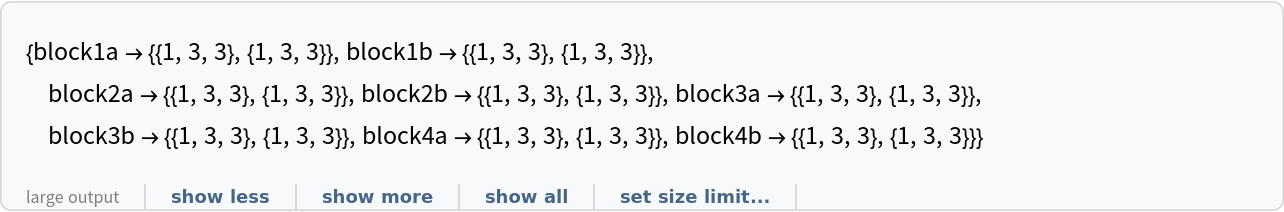The summary graphs for spatiotemporal ResNet-18 architectures of "(2+1)D", "3D" and "Mixed" models are presented below:

 Out=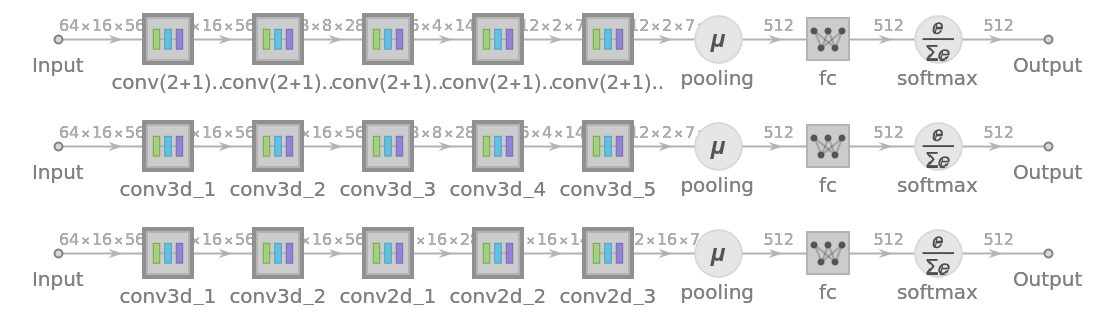Recommended evaluation of the network is time consuming:

 In:=Out=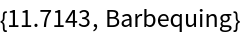Evaluation speed scales almost linearly with "TargetLength". The encoder can be tuned appropriately for speed vs. performance:

 In:=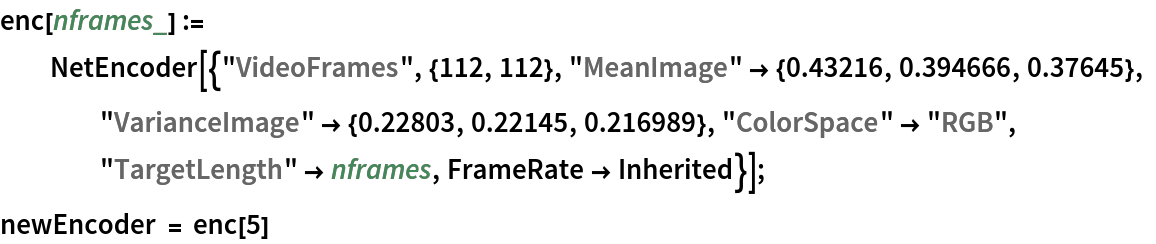Out=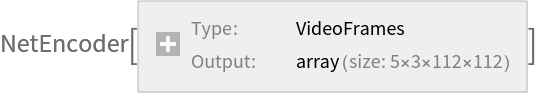Replace the encoder in the original NetModel:

 In:=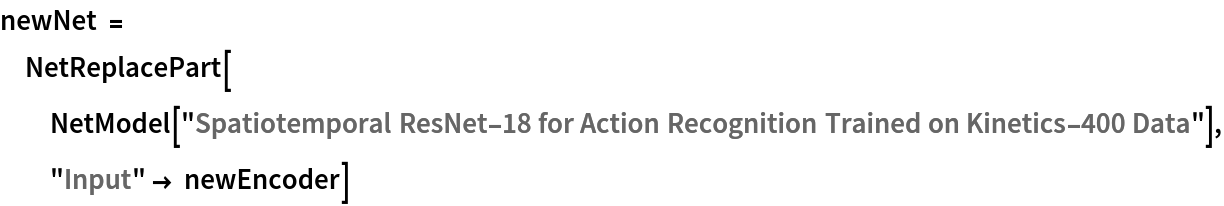Out=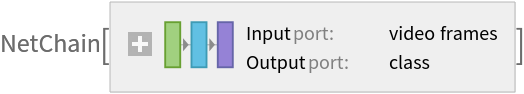Evaluate on the original video. The time of evaluation is reduced to a third, while the evaluation remains correct:

 In:=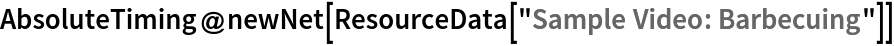Out=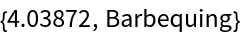### Feature extraction

Remove the last two layers of the trained net so that the net produces a vector representation of an image:

 In:=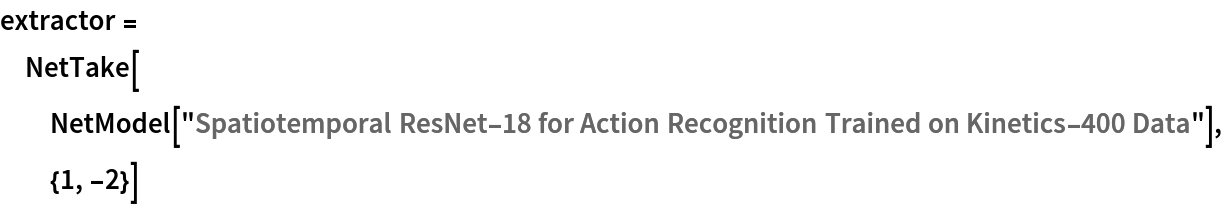Out=Get a set of videos:

 In:=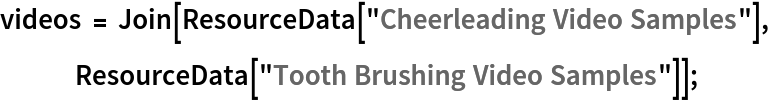Visualize the features of a set of videos:

 In:=Out=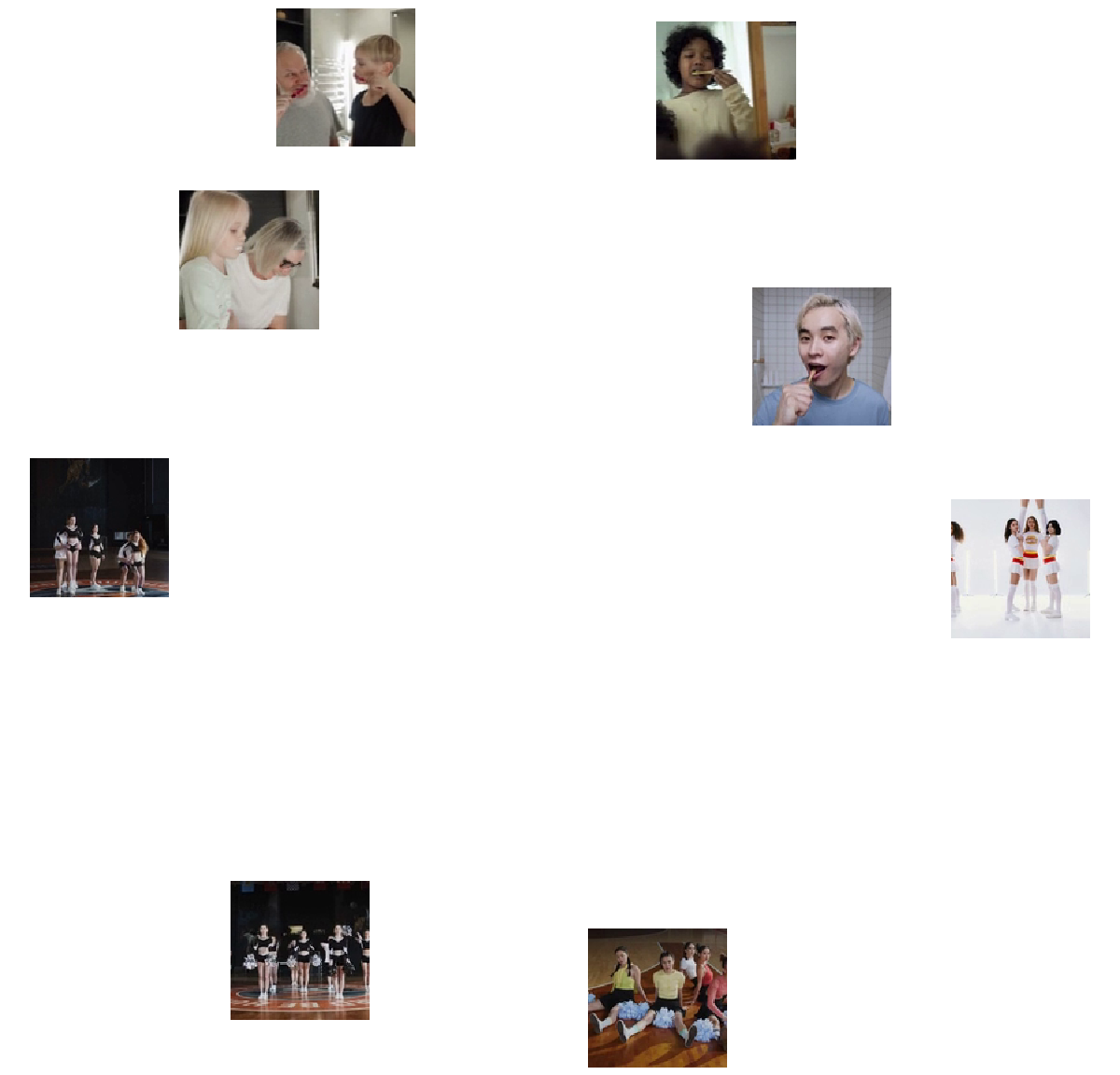### Net information

Inspect the number of parameters of all arrays in the net:

 In:=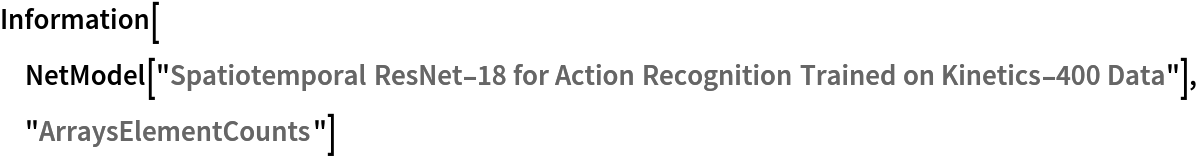Out=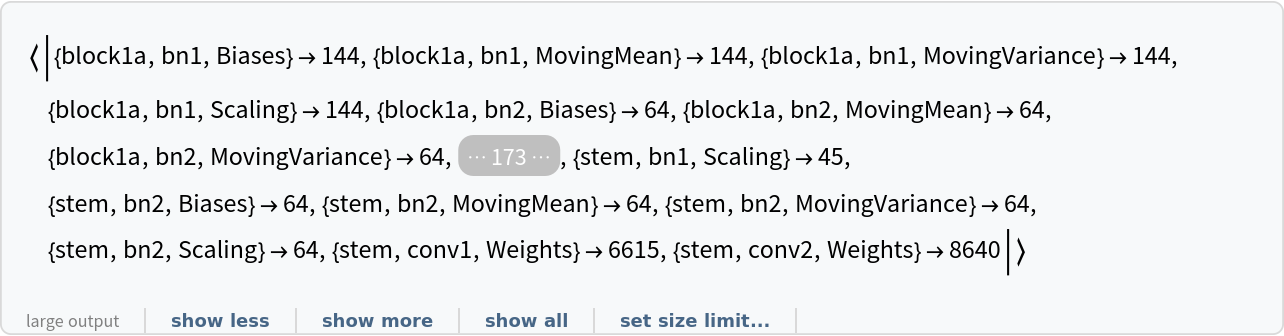Obtain the total number of parameters:

 In:=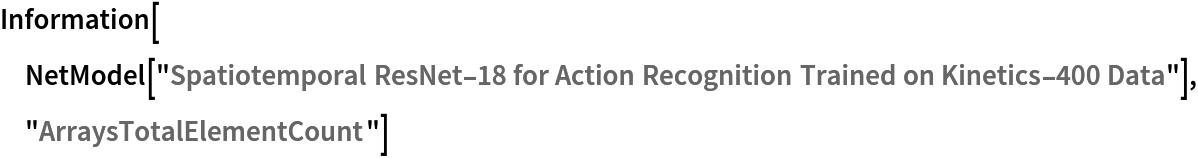Out=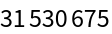Obtain the layer type counts:

 In:=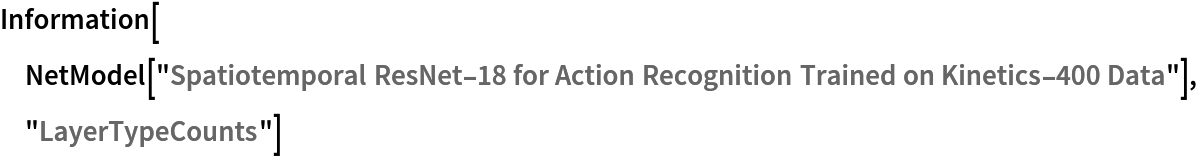Out=Display the summary graphic:

 In:=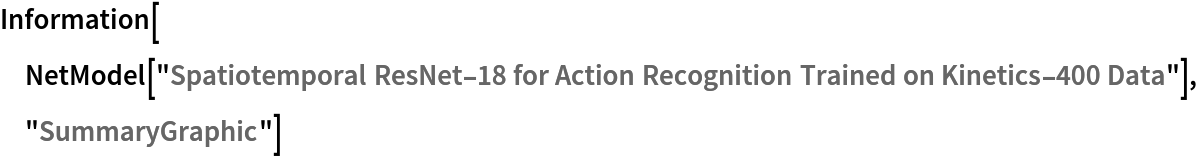Out=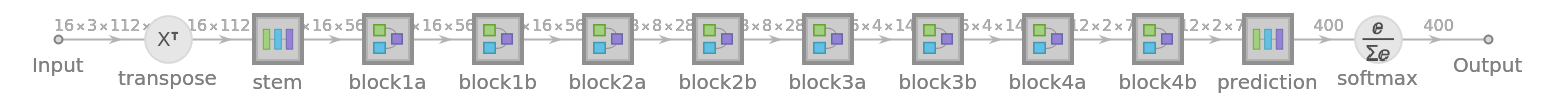### Export to ONNX

Export the net to the ONNX format:

 In:=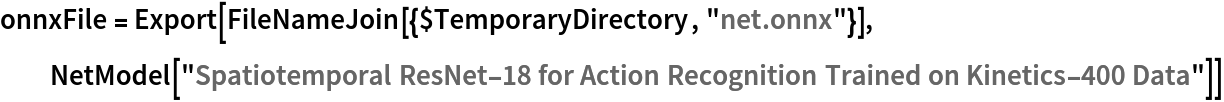Out=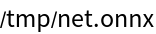Get the size of the ONNX file:

 In:=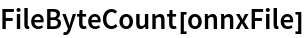Out=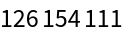The size is similar to the byte count of the resource object:

 In:=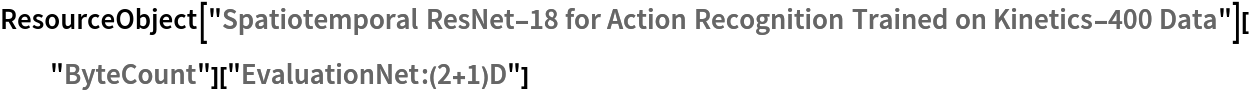Out=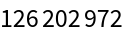Check some metadata of the ONNX model:

 In:=Out=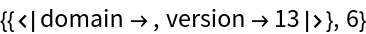Import the model back into the Wolfram Language. However, the NetEncoder and NetDecoder will be absent because they are not supported by ONNX:

 In:=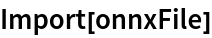Out=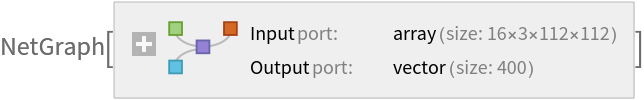## Reference

• D. Tran, H. Wang, L. Torresani, J. Ray, Y. LeCun, M. Paluri, "A Closer Look at Spatiotemporal Convolutions for Action Recognition," arXiv:1711.11248 (2018)